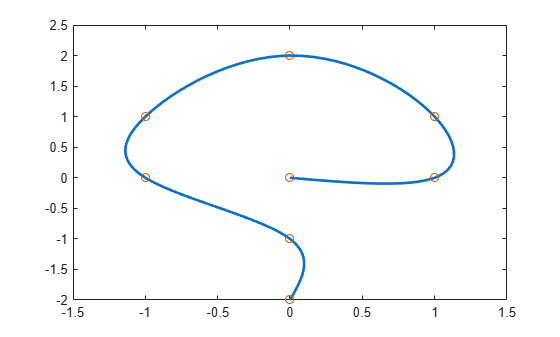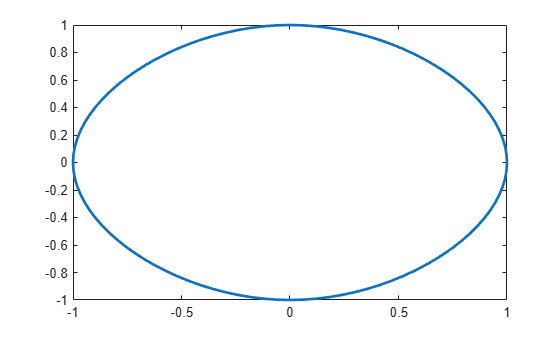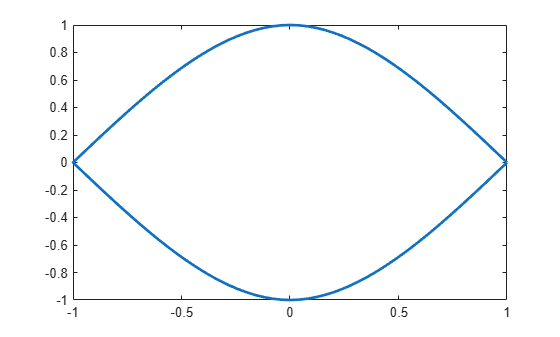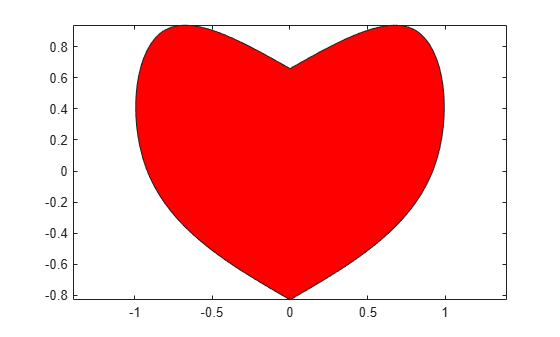# cscvn

“Natural” or periodic interpolating cubic spline curve

## Syntax

``curve = cscvn(points) ``

## Description

example

````curve = cscvn(points) ` returns a parametric variational, or natural, cubic spline curve (in ppform) passing through the given sequence points (:j), j = 1:end. The parameter value t(j) for the j-th point follows the Eugene Lee's  centripetal scheme, as accumulated square root of chord length:If the first and last point coincide and there are no other repeated points) then the function constructs a periodic cubic spline curve. However, double points result in corners.```

## Examples

collapse all

This example shows how to construct and plot several different interpolating cubic spline curves using the `cscvn` function.

This code generates a sequence of points and then plots the cubic spline generated from the cscvn function. The chosen points are marked as circles:

```points=[0 1 1 0 -1 -1 0 0; 0 0 1 2 1 0 -1 -2]; fnplt(cscvn(points)); hold on, plot(points(1,:),points(2,:),'o'), hold off```This code plots a circular curve through the four vertices of the standard diamond (because of the periodic boundary conditions enforced):

` fnplt(cscvn( [1 0 -1 0 1;0 1 0 -1 0] ))`This code shows a corner at the double point as well as at the curve endpoint:

` fnplt(cscvn( [1 0 -1 -1 0 1;0 1 0 0 -1 0] ))`Finally, this code generates a closed curve with one double point, which results in a corner. Dedicate this to your loved ones.

```c=fnplt(cscvn([0 .82 .92 0 0 -.92 -.82 0; .66 .9 0 ... -.83 -.83 0 .9 .66])); fill(c(1,:),c(2,:),'r'), axis equal```## Input Arguments

collapse all

Sequence of points at which the parametric "natural" cubic spline interpolates to, specified as a scalar, vector, or matrix.

## Output Arguments

collapse all

Spline in ppform, returned as a structure with these fields.

Form of the spline, returned as `pp`. `pp` indicates that the spline is given in piecewise polynomial form.

Knot positions of the spline, returned as a vector or as a cell array of vectors for multivariate data. Vectors contain strictly increasing elements that represent the start and end of each of the intervals over which the polynomial pieces are defined.

Coefficients of polynomials for each piece, returned as a matrix or as an array for multivariate data.

Number of polynomial pieces describing the spline, returned as a scalar or as a vector of numbers of pieces in each variable for multivariate data.

Order of the polynomial function describing each polynomial piece of the spline, returned as a scalar or as a vector containing the order in each variable for multivariate data.

Dimensionality of the target function, returned as a scalar.

## Algorithms

The function determines the break sequence `t` as

```t = cumsum([0;((diff(points.').^2)*ones(d,1)).^(1/4)]).'; ```

and uses `csape` (with either periodic or variational end conditions) to construct the smooth pieces between double points (if any).

 E. T. Y. Lee. “Choosing nodes in parametric curve interpolation.” Computer-Aided Design 21 (1989), 363–370.